Intermediate Algebra 2e

Intermediate Algebra 2e8.6 Solve Radical Equations

### Learning Objectives

By the end of this section, you will be able to:

### Be Prepared 8.16

Before you get started, take this readiness quiz.

Simplify: $(y−3)2.(y−3)2.$
If you missed this problem, review Example 5.31.

### Be Prepared 8.17

Solve: $2x−5=0.2x−5=0.$
If you missed this problem, review Example 2.2.

### Be Prepared 8.18

Solve $n2−6n+8=0.n2−6n+8=0.$
If you missed this problem, review Example 6.45.

In this section we will solve equations that have a variable in the radicand of a radical expression. An equation of this type is called a radical equation.

An equation in which a variable is in the radicand of a radical expression is called a radical equation.

As usual, when solving these equations, what we do to one side of an equation we must do to the other side as well. Once we isolate the radical, our strategy will be to raise both sides of the equation to the power of the index. This will eliminate the radical.

Solving radical equations containing an even index by raising both sides to the power of the index may introduce an algebraic solution that would not be a solution to the original radical equation. Again, we call this an extraneous solution as we did when we solved rational equations.

In the next example, we will see how to solve a radical equation. Our strategy is based on raising a radical with index n to the nth power. This will eliminate the radical.

$Fora≥0,(an)n=a.Fora≥0,(an)n=a.$

### Example 8.56

#### How to Solve a Radical Equation

Solve: $5n−4−9=0.5n−4−9=0.$

### Try It 8.111

Solve: $3m+2−5=0.3m+2−5=0.$

### Try It 8.112

Solve: $10z+1−2=0.10z+1−2=0.$

### How To

1. Step 1. Isolate the radical on one side of the equation.
2. Step 2. Raise both sides of the equation to the power of the index.
3. Step 3. Solve the new equation.
4. Step 4. Check the answer in the original equation.

When we use a radical sign, it indicates the principal or positive root. If an equation has a radical with an even index equal to a negative number, that equation will have no solution.

### Example 8.57

Solve: $9k−2+1=0.9k−2+1=0.$

### Try It 8.113

Solve: $2r−3+5=0.2r−3+5=0.$

### Try It 8.114

Solve: $7s−3+2=0.7s−3+2=0.$

If one side of an equation with a square root is a binomial, we use the Product of Binomial Squares Pattern when we square it.

### Binomial Squares

$(a+b)2=a2+2ab+b2(a−b)2=a2−2ab+b2(a+b)2=a2+2ab+b2(a−b)2=a2−2ab+b2$

Don’t forget the middle term!

### Example 8.58

Solve: $p−1+1=p.p−1+1=p.$

### Try It 8.115

Solve: $x−2+2=x.x−2+2=x.$

### Try It 8.116

Solve: $y−5+5=y.y−5+5=y.$

When the index of the radical is 3, we cube both sides to remove the radical.

$(a3)3=a(a3)3=a$

### Example 8.59

Solve: $5x+13+8=4.5x+13+8=4.$

### Try It 8.117

Solve: $4x−33+8=54x−33+8=5$

### Try It 8.118

Solve: $6x−103+1=−36x−103+1=−3$

Sometimes an equation will contain rational exponents instead of a radical. We use the same techniques to solve the equation as when we have a radical. We raise each side of the equation to the power of the denominator of the rational exponent. Since $(am)n=am·n,(am)n=am·n,$ we have for example,

$(x12)2=x,(x13)3=x(x12)2=x,(x13)3=x$

Remember, $x12=xx12=x$ and $x13=x3.x13=x3.$

### Example 8.60

Solve: $(3x−2)14+3=5.(3x−2)14+3=5.$

### Try It 8.119

Solve: $(9x+9)14−2=1.(9x+9)14−2=1.$

### Try It 8.120

Solve: $(4x−8)14+5=7.(4x−8)14+5=7.$

Sometimes the solution of a radical equation results in two algebraic solutions, but one of them may be an extraneous solution!

### Example 8.61

Solve: $r+4−r+2=0.r+4−r+2=0.$

### Try It 8.121

Solve: $m+9−m+3=0.m+9−m+3=0.$

### Try It 8.122

Solve: $n+1−n+1=0.n+1−n+1=0.$

When there is a coefficient in front of the radical, we must raise it to the power of the index, too.

### Example 8.62

Solve: $33x−5−8=4.33x−5−8=4.$

### Try It 8.123

Solve: $24a+4−16=16.24a+4−16=16.$

### Try It 8.124

Solve: $32b+3−25=50.32b+3−25=50.$

If the radical equation has two radicals, we start out by isolating one of them. It often works out easiest to isolate the more complicated radical first.

In the next example, when one radical is isolated, the second radical is also isolated.

### Example 8.63

Solve: $4x−33=3x+23.4x−33=3x+23.$

### Try It 8.125

Solve: $5x−43=2x+53.5x−43=2x+53.$

### Try It 8.126

Solve: $7x+13=2x−53.7x+13=2x−53.$

Sometimes after raising both sides of an equation to a power, we still have a variable inside a radical. When that happens, we repeat Step 1 and Step 2 of our procedure. We isolate the radical and raise both sides of the equation to the power of the index again.

### Example 8.64

#### How to Solve a Radical Equation

Solve: $m+1=m+9.m+1=m+9.$

### Try It 8.127

Solve: $3−x=x−3.3−x=x−3.$

### Try It 8.128

Solve: $x+2=x+16.x+2=x+16.$

We summarize the steps here. We have adjusted our previous steps to include more than one radical in the equation This procedure will now work for any radical equations.

### How To

1. Step 1. Isolate one of the radical terms on one side of the equation.
2. Step 2. Raise both sides of the equation to the power of the index.
3. Step 3. Are there any more radicals?
If yes, repeat Step 1 and Step 2 again.
If no, solve the new equation.
4. Step 4. Check the answer in the original equation.

Be careful as you square binomials in the next example. Remember the pattern is $(a+b)2=a2+2ab+b2(a+b)2=a2+2ab+b2$ or $(a−b)2=a2−2ab+b2.(a−b)2=a2−2ab+b2.$

### Example 8.65

Solve: $q−2+3=4q+1.q−2+3=4q+1.$

### Try It 8.129

Solve: $x−1+2=2x+6x−1+2=2x+6$

### Try It 8.130

Solve: $x+2=3x+4x+2=3x+4$

As you progress through your college courses, you’ll encounter formulas that include radicals in many disciplines. We will modify our Problem Solving Strategy for Geometry Applications slightly to give us a plan for solving applications with formulas from any discipline.

### How To

#### Use a problem solving strategy for applications with formulas.

1. Step 1. Read the problem and make sure all the words and ideas are understood. When appropriate, draw a figure and label it with the given information.
2. Step 2. Identify what we are looking for.
3. Step 3. Name what we are looking for by choosing a variable to represent it.
4. Step 4. Translate into an equation by writing the appropriate formula or model for the situation. Substitute in the given information.
5. Step 5. Solve the equation using good algebra techniques.
6. Step 6. Check the answer in the problem and make sure it makes sense.
7. Step 7. Answer the question with a complete sentence.

One application of radicals has to do with the effect of gravity on falling objects. The formula allows us to determine how long it will take a fallen object to hit the gound.

### Falling Objects

On Earth, if an object is dropped from a height of h feet, the time in seconds it will take to reach the ground is found by using the formula

$t=h4.t=h4.$

For example, if an object is dropped from a height of 64 feet, we can find the time it takes to reach the ground by substituting $h=64h=64$ into the formula.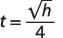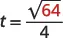Take the square root of 64.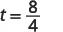Simplify the fraction.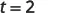It would take 2 seconds for an object dropped from a height of 64 feet to reach the ground.

### Example 8.66

Marissa dropped her sunglasses from a bridge 400 feet above a river. Use the formula $t=h4t=h4$ to find how many seconds it took for the sunglasses to reach the river.

### Try It 8.131

A helicopter dropped a rescue package from a height of 1,296 feet. Use the formula $t=h4t=h4$ to find how many seconds it took for the package to reach the ground.

### Try It 8.132

A window washer dropped a squeegee from a platform 196 feet above the sidewalk Use the formula $t=h4t=h4$ to find how many seconds it took for the squeegee to reach the sidewalk.

Police officers investigating car accidents measure the length of the skid marks on the pavement. Then they use square roots to determine the speed, in miles per hour, a car was going before applying the brakes.

### Skid Marks and Speed of a Car

If the length of the skid marks is d feet, then the speed, s, of the car before the brakes were applied can be found by using the formula

$s=24ds=24d$

### Example 8.67

After a car accident, the skid marks for one car measured 190 feet. Use the formula $s=24ds=24d$ to find the speed of the car before the brakes were applied. Round your answer to the nearest tenth.

### Try It 8.133

An accident investigator measured the skid marks of the car. The length of the skid marks was 76 feet. Use the formula $s=24ds=24d$ to find the speed of the car before the brakes were applied. Round your answer to the nearest tenth.

### Try It 8.134

The skid marks of a vehicle involved in an accident were 122 feet long. Use the formula $s=24ds=24d$ to find the speed of the vehicle before the brakes were applied. Round your answer to the nearest tenth.

### Media

Access these online resources for additional instruction and practice with solving radical equations.

### Section 8.6 Exercises

#### Practice Makes Perfect

In the following exercises, solve.

287.

$5 x − 6 = 8 5 x − 6 = 8$

288.

$4 x − 3 = 7 4 x − 3 = 7$

289.

$5x+1=−35x+1=−3$

290.

$3 y − 4 = −2 3 y − 4 = −2$

291.

$2 x 3 = −2 2 x 3 = −2$

292.

$4 x − 1 3 = 3 4 x − 1 3 = 3$

293.

$2 m − 3 − 5 = 0 2 m − 3 − 5 = 0$

294.

$2 n − 1 − 3 = 0 2 n − 1 − 3 = 0$

295.

$6 v − 2 − 10 = 0 6 v − 2 − 10 = 0$

296.

$12 u + 1 − 11 = 0 12 u + 1 − 11 = 0$

297.

$4 m + 2 + 2 = 6 4 m + 2 + 2 = 6$

298.

$6 n + 1 + 4 = 8 6 n + 1 + 4 = 8$

299.

$2 u − 3 + 2 = 0 2 u − 3 + 2 = 0$

300.

$5 v − 2 + 5 = 0 5 v − 2 + 5 = 0$

301.

$u − 3 + 3 = u u − 3 + 3 = u$

302.

$v − 10 + 10 = v v − 10 + 10 = v$

303.

$r − 1 = r − 1 r − 1 = r − 1$

304.

$s − 8 = s − 8 s − 8 = s − 8$

305.

$6 x + 4 3 = 4 6 x + 4 3 = 4$

306.

$11 x + 4 3 = 5 11 x + 4 3 = 5$

307.

$4 x + 5 3 − 2 = −5 4 x + 5 3 − 2 = −5$

308.

$9 x − 1 3 − 1 = −5 9 x − 1 3 − 1 = −5$

309.

$( 6 x + 1 ) 1 2 − 3 = 4 ( 6 x + 1 ) 1 2 − 3 = 4$

310.

$( 3 x − 2 ) 1 2 + 1 = 6 ( 3 x − 2 ) 1 2 + 1 = 6$

311.

$( 8 x + 5 ) 1 3 + 2 = −1 ( 8 x + 5 ) 1 3 + 2 = −1$

312.

$( 12 x − 5 ) 1 3 + 8 = 3 ( 12 x − 5 ) 1 3 + 8 = 3$

313.

$( 12 x − 3 ) 1 4 − 5 = −2 ( 12 x − 3 ) 1 4 − 5 = −2$

314.

$( 5 x − 4 ) 1 4 + 7 = 9 ( 5 x − 4 ) 1 4 + 7 = 9$

315.

$x + 1 − x + 1 = 0 x + 1 − x + 1 = 0$

316.

$y + 4 − y + 2 = 0 y + 4 − y + 2 = 0$

317.

$z + 100 − z = −10 z + 100 − z = −10$

318.

$w + 25 − w = −5 w + 25 − w = −5$

319.

$3 2 x − 3 − 20 = 7 3 2 x − 3 − 20 = 7$

320.

$2 5 x + 1 − 8 = 0 2 5 x + 1 − 8 = 0$

321.

$2 8 r + 1 − 8 = 2 2 8 r + 1 − 8 = 2$

322.

$3 7 y + 1 − 10 = 8 3 7 y + 1 − 10 = 8$

In the following exercises, solve.

323.

$3 u + 7 = 5 u + 1 3 u + 7 = 5 u + 1$

324.

$4 v + 1 = 3 v + 3 4 v + 1 = 3 v + 3$

325.

$8 + 2 r = 3 r + 10 8 + 2 r = 3 r + 10$

326.

$10 + 2 c = 4 c + 16 10 + 2 c = 4 c + 16$

327.

$5 x − 1 3 = x + 3 3 5 x − 1 3 = x + 3 3$

328.

$8 x − 5 3 = 3 x + 5 3 8 x − 5 3 = 3 x + 5 3$

329.

$2 x 2 + 9 x − 18 3 = x 2 + 3 x − 2 3 2 x 2 + 9 x − 18 3 = x 2 + 3 x − 2 3$

330.

$x 2 − x + 18 3 = 2 x 2 − 3 x − 6 3 x 2 − x + 18 3 = 2 x 2 − 3 x − 6 3$

331.

$a + 2 = a + 4 a + 2 = a + 4$

332.

$r + 6 = r + 8 r + 6 = r + 8$

333.

$u + 1 = u + 4 u + 1 = u + 4$

334.

$x + 1 = x + 2 x + 1 = x + 2$

335.

$a + 5 − a = 1 a + 5 − a = 1$

336.

$−2 = d − 20 − d −2 = d − 20 − d$

337.

$2 x + 1 = 1 + x 2 x + 1 = 1 + x$

338.

$3 x + 1 = 1 + 2 x − 1 3 x + 1 = 1 + 2 x − 1$

339.

$2 x − 1 − x − 1 = 1 2 x − 1 − x − 1 = 1$

340.

$x + 1 − x − 2 = 1 x + 1 − x − 2 = 1$

341.

$x + 7 − x − 5 = 2 x + 7 − x − 5 = 2$

342.

$x + 5 − x − 3 = 2 x + 5 − x − 3 = 2$

In the following exercises, solve. Round approximations to one decimal place.

343.

Landscaping Reed wants to have a square garden plot in his backyard. He has enough compost to cover an area of 75 square feet. Use the formula $s=As=A$ to find the length of each side of his garden. Round your answer to the nearest tenth of a foot.

344.

Landscaping Vince wants to make a square patio in his yard. He has enough concrete to pave an area of 130 square feet. Use the formula $s=As=A$ to find the length of each side of his patio. Round your answer to the nearest tenth of a foot.

345.

Gravity A hang glider dropped his cell phone from a height of 350 feet. Use the formula $t=h4t=h4$ to find how many seconds it took for the cell phone to reach the ground.

346.

Gravity A construction worker dropped a hammer while building the Grand Canyon skywalk, 4000 feet above the Colorado River. Use the formula $t=h4t=h4$ to find how many seconds it took for the hammer to reach the river.

347.

Accident investigation The skid marks for a car involved in an accident measured 216 feet. Use the formula $s=24ds=24d$ to find the speed of the car before the brakes were applied. Round your answer to the nearest tenth.

348.

Accident investigation An accident investigator measured the skid marks of one of the vehicles involved in an accident. The length of the skid marks was 175 feet. Use the formula $s=24ds=24d$ to find the speed of the vehicle before the brakes were applied. Round your answer to the nearest tenth.

#### Writing Exercises

349.

Explain why an equation of the form $x+1=0x+1=0$ has no solution.

350.

Solve the equation $r+4−r+2=0.r+4−r+2=0.$ Explain why one of the “solutions” that was found was not actually a solution to the equation.

#### Self Check

After completing the exercises, use this checklist to evaluate your mastery of the objectives of this section.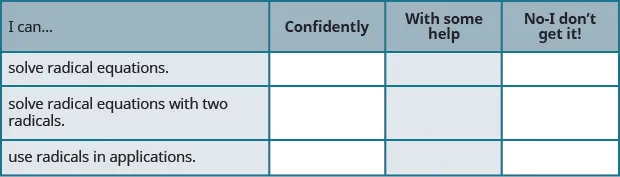After reviewing this checklist, what will you do to become confident for all objectives?

Order a print copy

As an Amazon Associate we earn from qualifying purchases.